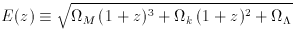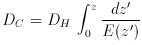### 4. COMOVING DISTANCE (LINE-OF-SIGHT)

A small comoving distanceDC between two nearby objects in the Universe is the distance between them which remains constant with epoch if the two objects are moving with the Hubble flow. In other words, it is the distance between them which would be measured with rulers at the time they are being observed (the proper distance) divided by the ratio of the scale factor of the Universe then to now. In other words the proper distance multiplied by (1 + z). The total line-of-sight comoving distance DC from us to a distant object is computed by integrating the infinitesimalDC contributions between nearby events along the radial ray from z = 0 to the object.

Following Peebles (1993, pp. 310-321) (who calls the transverse comoving distance by the confusing name ``angular size distance,'' which is not the same as ``angular diameter distance'' introduced below), we define the function(13)

which is proportional to the time derivative of the logarithm of the scale factor (ie,(t) / a (t)), with z redshift and the three density parameters defined as above. (For this reason, H (z) = H0 E (z) is the Hubble constant as measured by a hypothetical astronomer working at redshift z.) Since dz = da, dz / E (z) is proportional to the time-of-flight of a photon traveling across the redshift interval dz, divided by the scale factor at that time. Since the speed of light is constant, this is a proper distance divided by the scale factor, which is the definition of a comoving distance. The total line-of-sight comoving distance is then given by integrating these contributions, or(14)

where DH is the Hubble distance defined above.

In some sense the line-of-sight comoving distance is the fundamental distance measure in cosmography since, as will be seen below, all others are quite simply derived in terms of it. The line-of-sight comoving distance between two nearby events (ie, close in redshift or distance) is the distance which we would measure locally between the events today if those two points were locked into the Hubble flow. It is the correct distance measure for measuring aspects of large-scale structure imprinted on the Hubble flow, eg, distances between ``walls.''